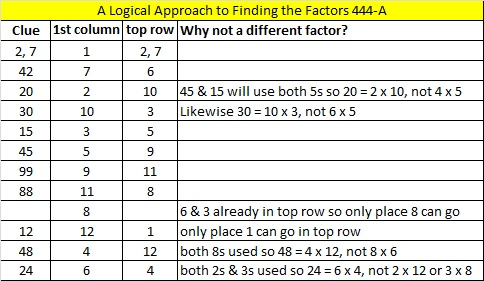# 287 and Level 4

• 287 is a composite number.
• Prime factorization: 287 = 7 x 41
• The exponents in the prime factorization are 1 and 1. Adding one to each and multiplying we get (1 + 1)(1 + 1) = 2 x 2 = 4. Therefore 287 has 4 factors.
• Factors of 287: 1, 7, 41, 287
• Factor pairs: 287 = 1 x 287 or 7 x 41
• 287 has no square factors that allow its square root to be simplified. √287 ≈ 16.941.Here’s today’s puzzle:Print the puzzles or type the factors on this excel file: 12 Factors 2014-11-03This site uses Akismet to reduce spam. Learn how your comment data is processed.